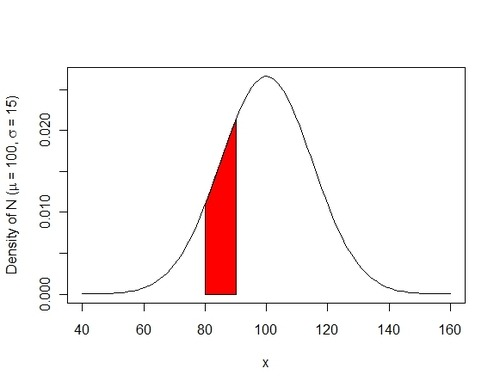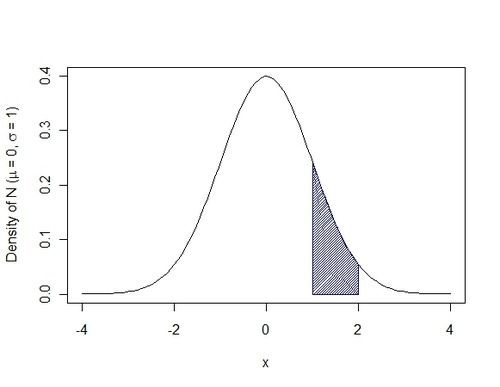Want to share your content on R-bloggers? click here if you have a blog, or here if you don't.

The problem: We often want to plot data and assign plot attributes based on characteristics of the data. For example, if we have a group of students with the following IQs, we might want to indicate who is an outlier in the statistical sense. I like using the IQ measure as an example for this, even though I think the measure itself is imperfect, as it has nice properties characteristic of the normal distribution.

So, IQ $$\sim N( \mu= 100, \sigma = 15)$$

We can plot this using:

Now, what if we want to visualize how much of that distribution falls between two points? Well, we may want to shade in the area under the curve along some interval (A, B). Next we’d want to tell R to fill that area of the curve with a color. But, you may ask, what if the parameters of interest (our interval, population mean, standard deviation, etc.) change? Would we have to re-do all of our plotting? No; not if we write a short program to do what we described above for any set of inputs: our population mean(MU), the standard deviation (SD) of the population, the interval, defined by (Lower, Upper) points the color we want to fill the plot with. Luckily, with basic programming, this is relatively pain-free to do. Below is a function, called shade.norm that takes five arguments (MU, SD, Lower, Upper, Color) and produces a plot in base graphics to answer our question, shown here:If this is your first function, you should know that functions are defined by setting the arguments R should look for, as well as what R should do with those arguments. Our function takes five arguments (MU, SD, Lower, Upper, Color) and next makes our cord.x vector which contains a sequence (seq()) of a large number of points between our Lower and Upper bounds; Next, it makes a cord.y vector that contains the heights of the normal density defined by MU and SD; third, using the curve() command, we plot a curve of the density of the normal(MU, SD) and make the x.axis limits run to +/- four standard deviations, essentially covering 100% of the visible area below the curve. Finally, we add a polygon whose vertices are defined by those vectors, and we specify the color for R to fill in.  The color you use for fill, to keep the program simple, should appear in the function call as in single quotes, e.g. ‘red’  The Y.axis text shows a hackish way of using the bquote command to combine Greek letters and variables into a useable plot label. Extra space was added, using * ” ” * for aesthetic purposes. For help, see ?bquote

Now, you can customize this to fit any type of shading problem you encounter. Even if the plots seem trivial, they may help both the beginning statistician as well as the new useR. If nothing else, you can see how to combine Greek/Math in a plot title along with a variable. That alone might be useful. An example of the final shading problem, with shading density=45 is below:

shade.norm.density(0, 1, 1, 2, ‘darkblue’, 45)